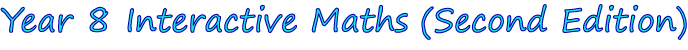1. Arithmetic Revision
2. Fractions and Decimals
3. Integers
4. Algebra
5. Equations
6. Ratios
7. Indices
8. Consumer Arithmetic
9. Reasoning in Geometry
10. Geometric Constructions
11. Length and Perimeter
12. Area of Plane Figures
13. Volume
14. Rates
15. Linear Graphs
16. Probability
17. Statistics
18. RevisionThe Year 8 Interactive Maths software is compatible with both Windows® and Mac® computers.  Discover more about Year 8 Interactive Maths by:

• Viewing the 17 chapter screen shots shown below that show step-by-step solutions in G S Rehill's proven writing style.  Students use the solutions after answering questions from the interactive exercises to identify mistakes in their working and to reinforce concepts, reasoning or mathematical laws.
• Clicking Mathematics Software Tutorials to watch 2 tutorials that show how students use the mathematics software.From Chapter 1: Arithmetic Revision, Exercise 1: Addition of Large Numbers.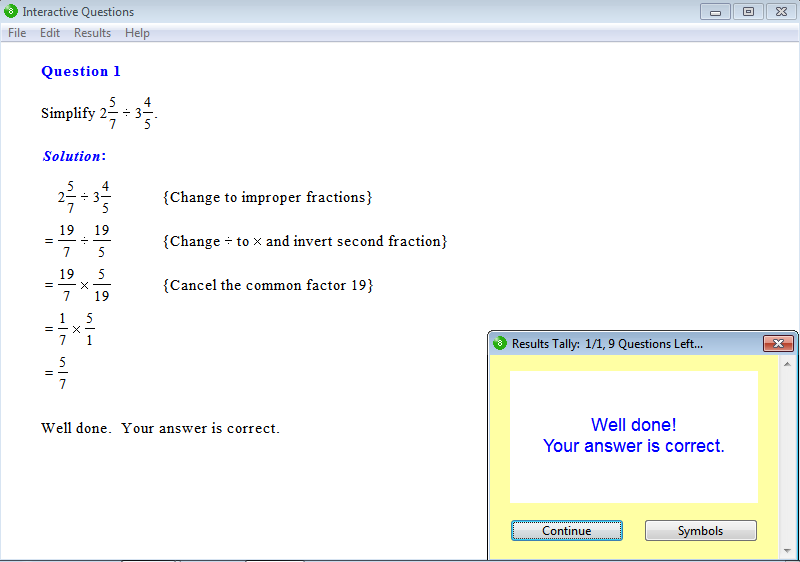From Chapter 2:  Fractions and Decimals, Exercise 11: Division of Fractions.From Chapter 3: Integers, Exercise 4: Integers.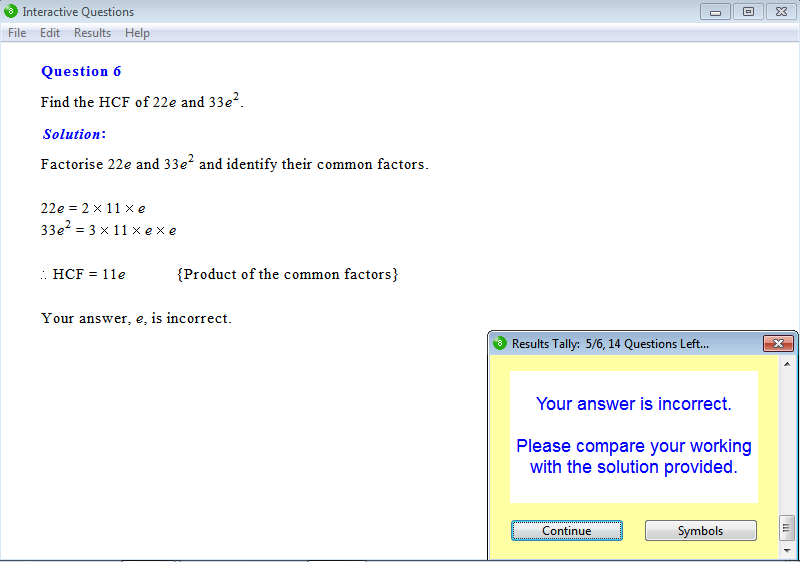From Chapter 4: Algebra, Exercise 21: Highest Common Factor of Algebraic Expressions.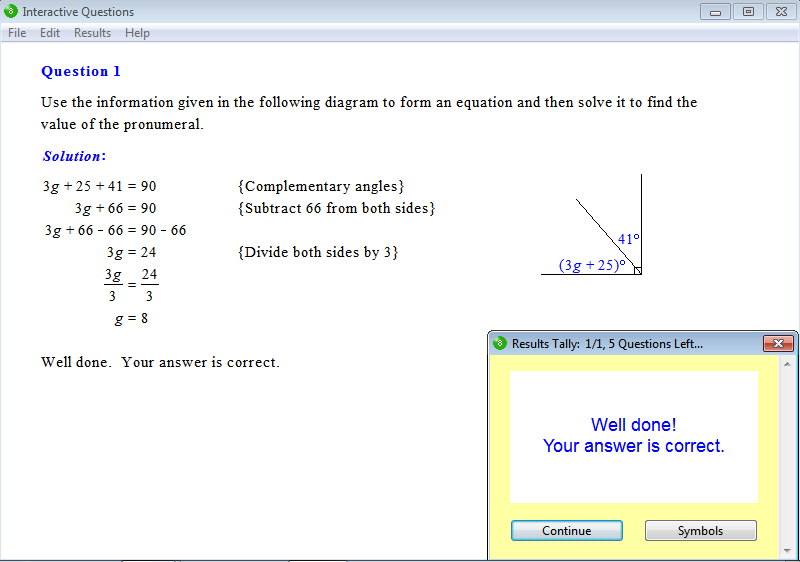From Chapter 5: Equations, Exercise 24: Problem Solving.From Chapter 6: Ratios, Exercise 6: Finding the Ratio of Two Quantities.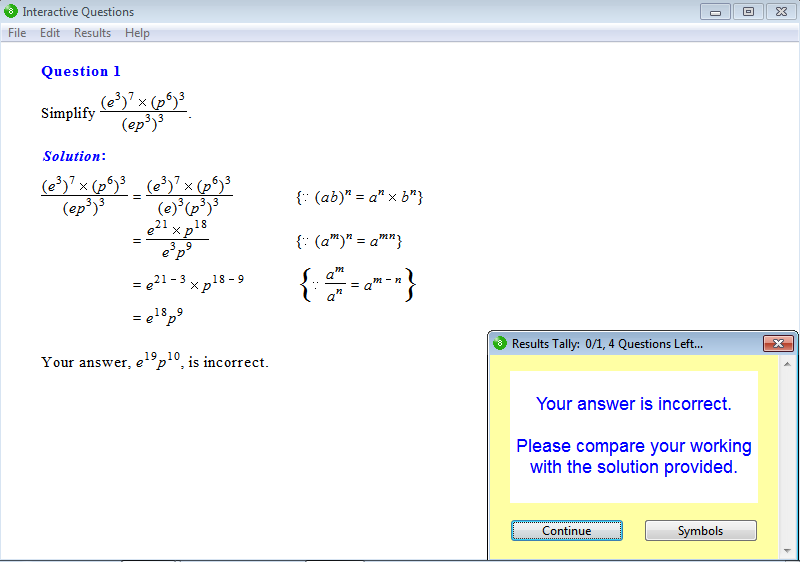From Chapter 7: Indices, Exercise 22: Index Law for Powers of Products.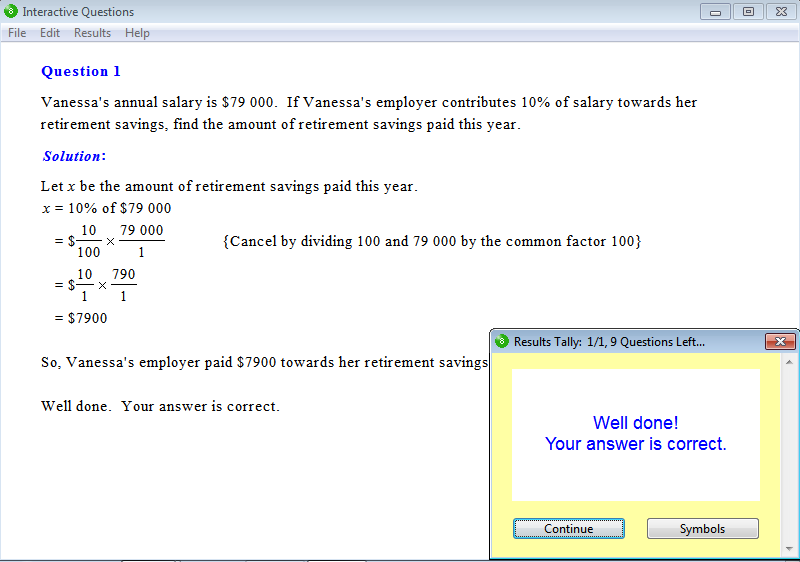From Chapter 8: Consumer Arithmetic, Exercise 11: Finding a Percentage of a Quantity.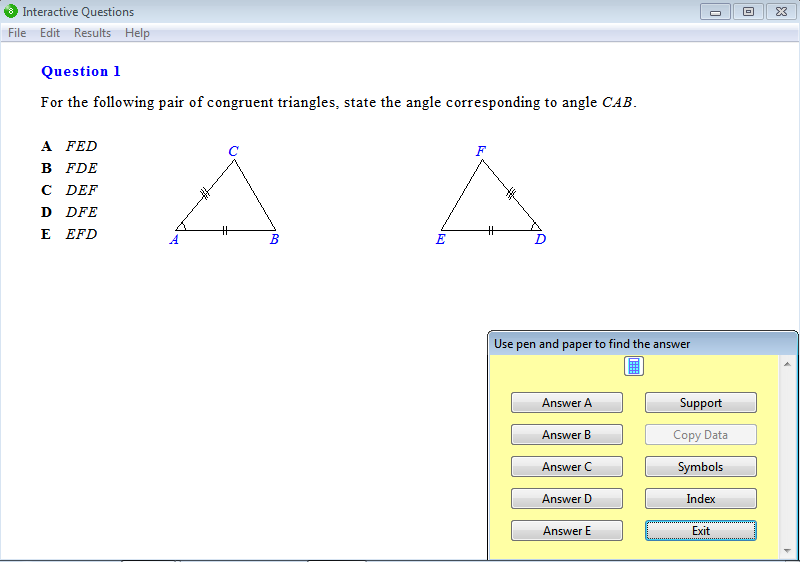From Chapter 9: Reasoning in Geometry, Exercise 16: Congruent Triangles.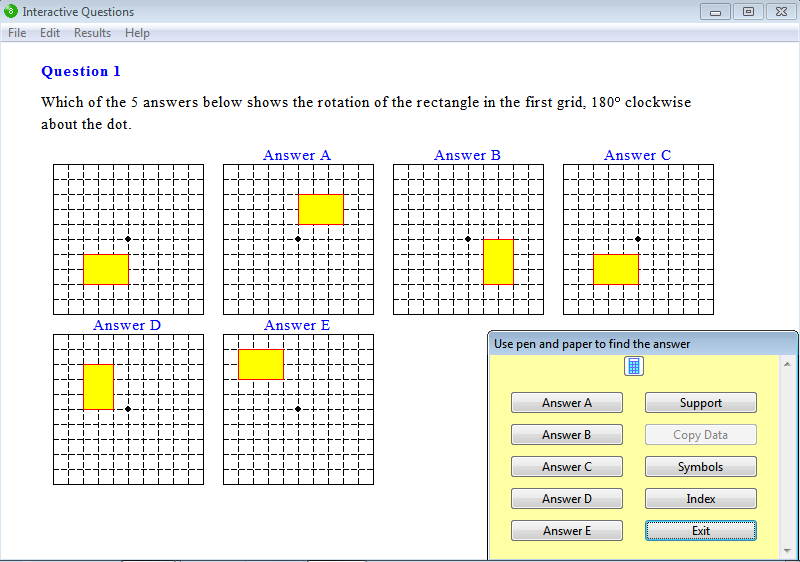From Chapter 10: Geometric Constructions, Exercise 6: Rotation.From Chapter 11: Length and Perimeter, Exercise 6: Word Problems.From Chapter 12: Area of Plane Figures, Exercise 15: Area of a Composite Figure.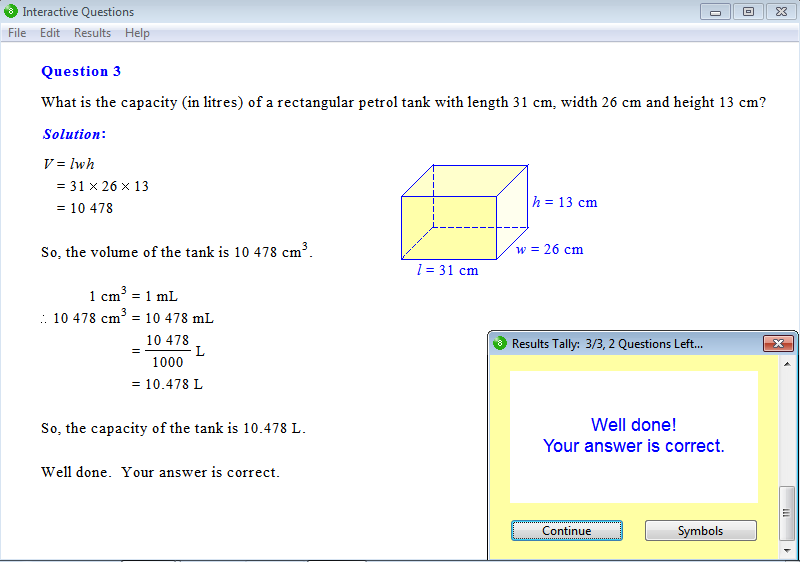From Chapter 13: Volume, Exercise 11: Capacity.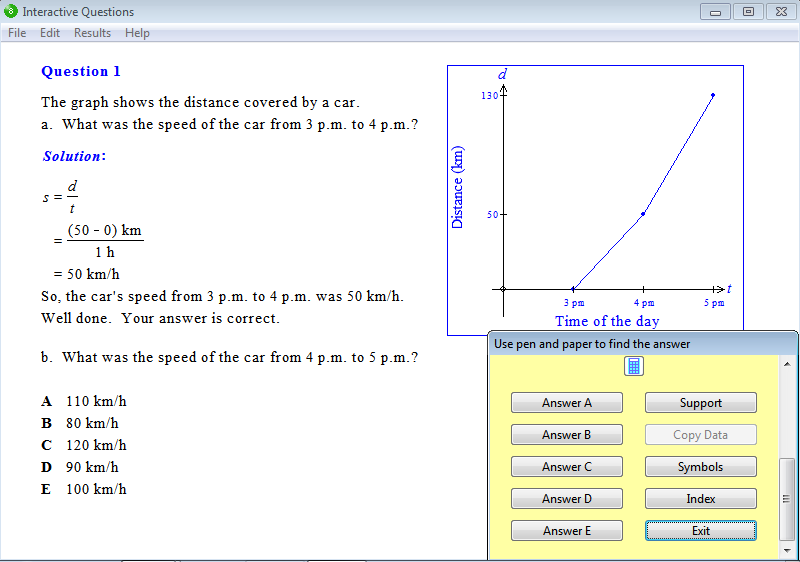From Chapter 14: Rates, Exercise 9: Travel Graphs.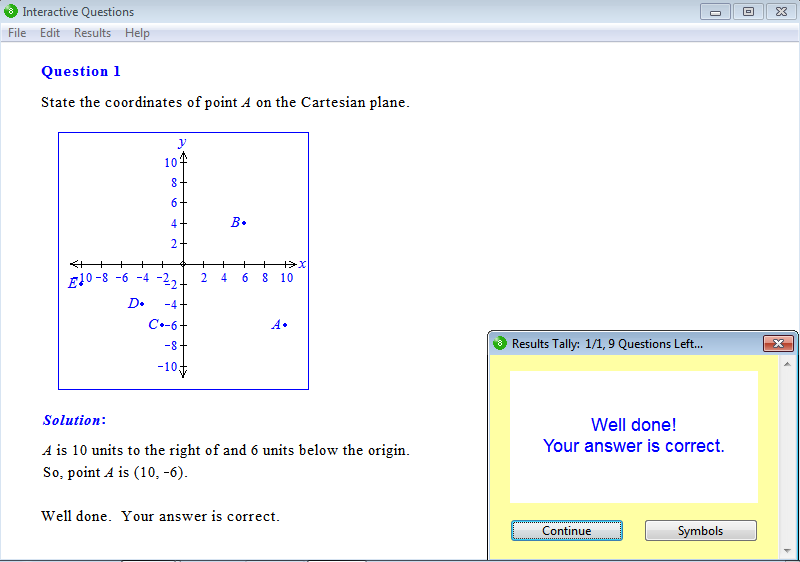From Chapter 15: Linear Graphs, Exercise 1: The Cartesian Plane.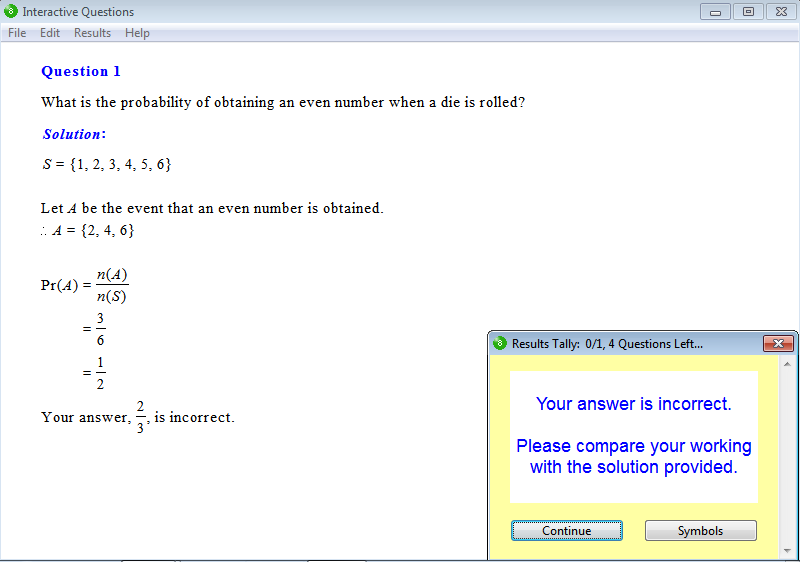From Chapter 16: Probability, Exercise 3: Probability.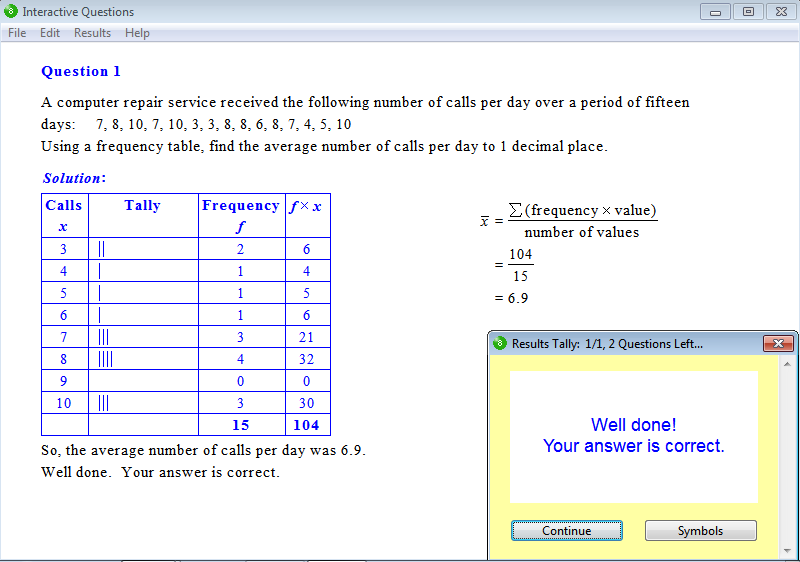From Chapter 17: Statistics, Exercise 4: Frequency Tables and the Mean.

Parents can order a 12-month Homework Licence for \$19.95 (AUD) per student by clicking this order button: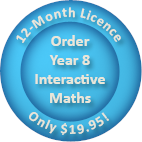Tutors may order Tutor Licences online that feature the interactive exercises as well as worksheets, tests and solution sheets.